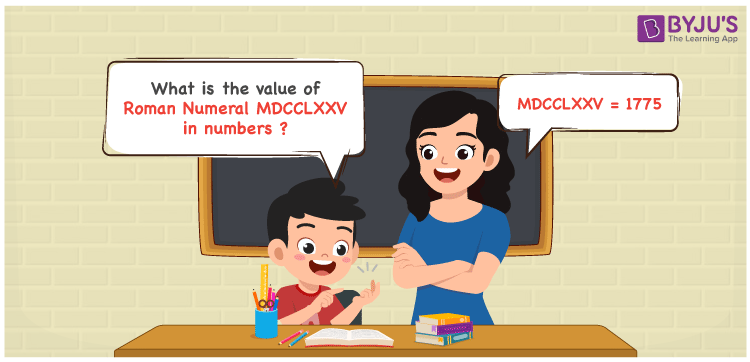Checkout JEE MAINS 2022 Question Paper Analysis : Checkout JEE MAINS 2022 Question Paper Analysis :

# MDCCLXXV Roman Numerals

MDCCLXXV Roman Numerals is 1775. This article has the steps used to transform the Roman numerals MDCCLXXV to numbers to assist students in the learning process. The roman numerals conversion article clears all your doubts immediately and speeds up your exam preparation. Therefore, the roman numerals MDCCLXXV is written as 1775 in numbers.

 Number Roman Numeral 1775 MDCCLXXV## How to Write MDCCLXXV Roman Numerals in Numbers?

This section shows how MDCCLXXV is split and converted to numbers using the symbols chart.

MDCCLXXV = M + D + C + C + L + X + X + V

MDCCLXXV = 1000 + 500 + 100 + 100 + 50 + 10 + 10 + 5

MDCCLXXV = 1775

## Video Lesson on Roman Numerals## Frequently Asked Questions on MDCCLXXV Roman Numerals

### Why is MDCCLXXV the roman numerals of 1775?

We know that

M is the roman numerals of 1000

DCC is the roman numerals of 700

LXX is the roman numerals of 70

V is the roman numerals of 5

So MDCCLXXV is the roman numeral of 1775.

### Determine 1800 – 25.

We know that

1800 – 25 = 1775

Hence, 1800 – 25 is 1775 which is written as MDCCLXXV.

### Find the remainder if MDCCLXXV is divided by XV.

We know that

MDCCLXXV = 1775

XV = 15

The remainder if MDCCLXXV is divided by XV is V.